Positive derivative at a point not implies increasing around the point

Statement

It is possible to have a continuous function (in fact, even a differentiable function)$f: \R \to \R$ and a point$x_0$ such that$f'(x_0)$ is positive, but there is no$\varepsilon > 0$ such that$f$ is an increasing function on$(x_0 - \varepsilon, x_0 + \varepsilon)$.

Facts used

1. Negative derivative implies decreasing

Proof

Example involving sine of reciprocal function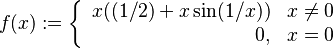$f(x) := \left\lbrace \begin{array}{rl} x((1/2) + x \sin(1/x)) & x\ne 0 \\ 0, & x = 0 \\\end{array}\right.$

Continuity and derivative at zero (equals 1/2)

The limit at zero is: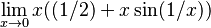$\lim_{x \to 0} x((1/2) + x \sin(1/x))$

We break this up: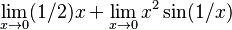$\lim_{x \to 0} (1/2)x + \lim_{x \to 0} x^2 \sin(1/x)$

The first limit goes to zero by continuity. The second limit works out to zero because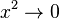$x^2 \to 0$ and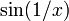$\sin(1/x)$ is bounded.

We therefore get a limit of zero, proving continuity.

The derivative at zero is: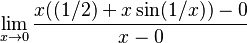$\lim_{x \to 0} \frac{x((1/2) + x \sin(1/x)) - 0}{x - 0}$

We can cancel$x$ giving:$\lim_{x \to 0} ((1/2) + x \sin(1/x))$

The 1/2 can be pulled out: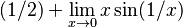$(1/2) + \lim_{x \to 0} x \sin (1/x)$

We have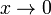$x \to 0$ and$\sin(1/x)$ is bounded, so the limit expression goes to zero.

We therefore get a derivative at 0 with value (1/2).

Derivative around zero

For$x \ne 0$, the derivative can be calculated formally by differentiating the expression for$f$. We get:$f'(x) = (1/2) + 2x \sin (1/x) - \cos(1/x)$

The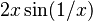$2x \sin (1/x)$ portion of the expression approaches zero as$x \to 0$, but$\cos(1/x)$ oscillates in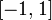$[-1,1]$ arbitrarily close to zero. Therefore,$f'$ takes all values in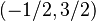$(-1/2,3/2)$ for x[/itex] arbitrarily close to zero (note that we can't (easily) close the interval because of the complicating effect of$2x \sin(1/x)$).

Implications for increasing/decreasing

In particular, this means that$f'$ takes negative values for$x$ arbitrarily close to 0. Moreover, since it's continuous everywhere except zero, it takes negative values over subintervals inside any open interval about 0. On these subintervals, therefore, since negative derivative implies decreasing,$f$ is decreasing. And therefore,$f$ cannot be increasing on the whole interval.

Thinking to motivate this example

If$f'$ were continuous at$x_0$, then$f'$ must be positive in some open interval around$x_0$. On that open interval, positive derivative implies increasing would force$f$ to be increasing.

Therefore, any counterexample must have$f'$ not continuous at$x_0$. We therefore use the example function for derivative of differentiable function need not be continuous as our starting point. The problem with this function (for our purposes) is that the derivative at the point of interest is zero. To get a positive derivative, we add the simplest function with constant positive derivative: a scalar multiple function.

The next question is: what scalar multiple to use? If we chose a coefficient (i.e., slope) greater than 1, then, even though the derivative is not continuous, we will still end up getting that the derivative is positive everywhere (because the variation to the derivative is bounded by 1). Even a slope of 1 gets us to nonnegative derivative everywhere. We really need a slope between 0 and 1 to allow the derivative to fluctuate sign arbitrarily close to zero. That's how to get our example.